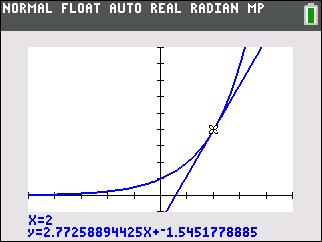# Activities

••• ##### Subject Area

• Math: Algebra II: Logarithms and Exponentials

• ##### Author9-12

45 Minutes

• ##### Device
• TI-83 Plus Family
• TI-84 Plus
• TI-84 Plus Silver Edition
•TI-84 Plus C Silver Edition
•TI-84 Plus CE
• ##### Report an Issue

Exponential Growth#### Activity Overview

Students will find an approximation for the value of the mathematical constant e and to apply it to exponential growth and decay problems.

#### Key Steps

•In Problem 1, students investigate the how the value of b affects the shape of the graph y = bx.

•In Problem 2, students use a line tangent to the curve at a point to explore the relationship between the slope of the tangent line at that point and the value of the function at the same point.

•At the end of this activity, students will discover that there is exactly one value of b, Euler’s number, for which the slope of the tangent and value of the function are equal.

Students will also explore and model applications of exponential growth in given situations.